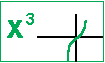## Polynomial Functions

• To provide a list of things to do when sketching a polynomial function,
• To provide an example of sketching polynomial functions,
• To give you a problems to try and their solution, and
• To assign related questionbook questions on this topic to be submitted either through email or through the folder on my desk.

You might wish to review how to take notes on a computer.

 To sketch a polynomial function: · Note the degree of the polynomial -- use it to predict the general shape and end behavior. -- even functions "start high & end high" -- odd functions "start low & end high"· Note the coefficient of the term with highest degree -- use it to determine if the curve is reflected about the x-axis.   x³ "starts low and ends high"       (generally increases as x increases)   - x³ "starts high and ends low"       (generally decreases as x increases)· Rewrite it by factoring identify the linear, quadratic, or other factors. · Plot the real zeros. · Note for each root or zero what kind of a root it is -- odd powers pass through the x-axis, -- even powers touch but do not pass through the x-axis.· Plot (0, f(0)), the y-intercept. · Solve: first derivative = 0 -- to find relative maximums/minimums. · Determine sign in intervals -- using the positiveness or negativeness of each factor. -- just "connect the dots." · Sketch curve.

 An Example of the Sketching Polynomial Functions     See the computer sketchor go step-by-step through the sketching of this polynomial.     Use your browser to return to this page.

 Try these.     1. Sketch and labelthen check the answer.     2. Sketch and labelthen check the answer.

 Question 19, 20 19.   Decompose, factor, the polynomial. 19a. State the linear factors. 19b. State the quadratic factors. 19c. State the end behavior of the function when         x approaches negative infinity. 19d. State the end behavior of the function when         x approaches positive infinity. 19e. Sketch the graph.   20.   Create and state your own polynomial function         with the following characteristics. It has a double root at -3. It has a pair of imaginary roots. It has an x-intercept at 6. It has a single root at 4.

Return to: Dilation by a constant   Dilation   Polynomial Functions   Rational Functions
 © 1999, 2/23/04, 2010, A2 mathnstuff.com/math/spoken/here/2class/300/fx/poly.htm